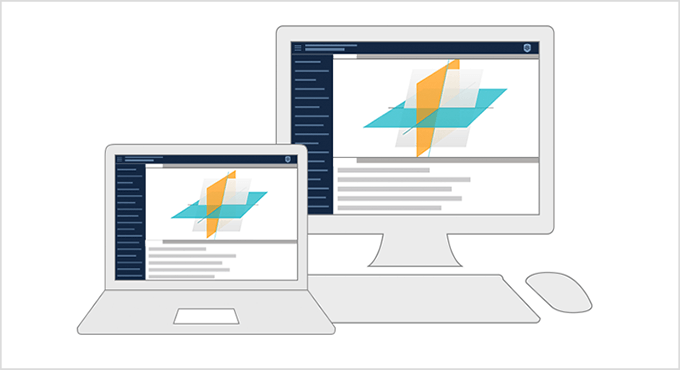#Wolfram U

Interactive Course

# Introduction to Linear Algebra

4hr 30min
Intermediate
2 Certifications
Estimated Time: 4hr 30min
Course Level: Intermediate
Prerequisites: This course requires no prior knowledge of Mathematica or the Wolfram Language. Prerequisites for linear algebra include algebra, elementary geometry and trigonometry.
Certification Levels: Course completion, Wolfram Certified Level I### Summary

A comprehensive introduction to fundamental concepts in linear algebra, including video lessons and interactive notebooks. Follow along with the examples in the Wolfram Cloud and use the material to prepare for courses in data science, engineering and other fields. The course starts with linear equations and matrices, followed by determinants and eigenvalues, and then moves on to inner products and the singular value decomposition. Application sessions are included to show uses of linear algebra in the real world. Exercises and quizzes are provided for self-paced assessment. Earn a certificate of course completion by watching all lesson and application session videos and completing the quizzes with a passing grade. Level I certification in linear algebra is awarded to those who meet the completion requirements and also pass the course final exam.

### You'll Learn To

Solve linear systems of equations

Compute products and inverses of matrices

Use determinants to solve linear systems

Work with eigenvalues and eigenvectors

Calculate least-squares approximations

Apply the singular value decompositionAsk questions or start a discussion about related topics on Wolfram Community.